## Saturday, 9 August 2014

### chapter 7 Some Standard Limits 3

(G)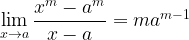$\mathop {\lim }\limits_{x \to a} \dfrac{{{x^m} - {a^m}}}{{x - a}} = m{a^{m - 1}}$
This is an extension of the previous limit as follows: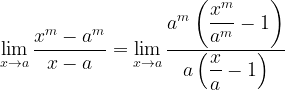$\mathop {\lim }\limits_{x \to a} \dfrac{{{x^m} - {a^m}}}{{x - a}} = \mathop {\lim }\limits_{x \to a} \dfrac{{{a^m}\left( {\dfrac{{{x^m}}}{{{a^m}}} - 1} \right)}}{{a\left( {\dfrac{x}{a} - 1} \right)}}$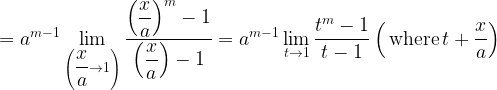$= {a^{m - 1}}\mathop {\lim }\limits_{\left( {\dfrac{x}{a} \to 1} \right)} \dfrac{{{{\left( {\dfrac{x}{a}} \right)}^m} - 1}}{{\left( {\dfrac{x}{a}} \right) - 1}} = {a^{m - 1}}\mathop {\lim }\limits_{t \to 1} \dfrac{{{t^m} - 1}}{{t - 1}}\left( \,{{\rm{where}}\,t + \dfrac{x}{a}} \right)$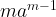$m{a^{m - 1}}$
(H)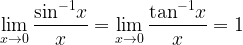$\mathop {\lim }\limits_{x \to 0} \dfrac{{{{\sin }^{ - 1}}x}}{x} = \mathop {\lim }\limits_{x \to 0} \dfrac{{{{\tan }^{ - 1}}x}}{x} = 1$
We have seen the limits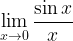$\mathop {\lim }\limits_{x \to 0} \dfrac{{\sin x}}{x}$ and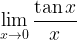$\mathop {\lim }\limits_{x \to 0} \dfrac{{\tan x}}{x}$ .
How can we use these limits to derive the limits that we require now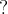$?$
Consider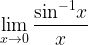$\mathop {\lim }\limits_{x \to 0} \dfrac{{{{\sin }^{ - 1}}x}}{x}$ . Substituting${\sin ^{ - 1}}x = t$ gives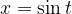$x = \sin t$. Also, as$x \to 0,{\sin ^{ - 1}}x \to 0\,{\rm{or}}\,t \to 0.$
Hence, our limit reduces to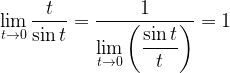$\mathop {\lim }\limits_{t \to 0} \dfrac{t}{{\sin t}} = \dfrac{1}{{\mathop {\lim }\limits_{t \to 0} \left( {\dfrac{{\sin t}}{t}} \right)}} = 1$
Similarly,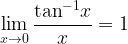$\mathop {\lim }\limits_{x \to 0} \dfrac{{{{\tan }^{ - 1}}x}}{x} = 1$

Having seen some important indeterminate limits, notice the following expansions that can be used to evaluate limits.Evaluate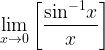$\mathop {\lim }\limits_{x \to 0} \left[ {\dfrac{{{{\sin }^{ - 1}}x}}{x}} \right]$ and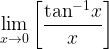$\mathop {\lim }\limits_{x \to 0} \left[ {\dfrac{{{{\tan }^{ - 1}}x}}{x}} \right]$
 (a)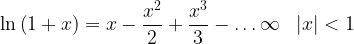$\ln \left( {1 + x} \right) = x - \dfrac{{{x^2}}}{2} + \dfrac{{{x^3}}}{3} - \ldots\infty \,\,\,\,\left| x \right| < 1$ (b)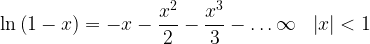$\ln \left( {1 - x} \right) = - x - \dfrac{{{x^2}}}{2} - \dfrac{{{x^3}}}{3} - \ldots\infty \,\,\,\, \left| x \right| < 1$ (c)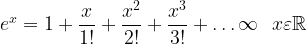${e^x} = 1 + \dfrac{x}{{1!}} + \dfrac{{{x^2}}}{{2!}} + \dfrac{{{x^3}}}{{3!}} + \ldots\infty \,\,\,\, x\varepsilon\mathbb{R}$ (d)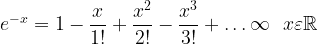${e^{ - x}} = 1 - \dfrac{x}{{1!}} + \dfrac{{{x^2}}}{{2!}} - \dfrac{{{x^3}}}{{3!}} + \ldots\infty \,\,\,\, x\varepsilon\mathbb{R}$ (e)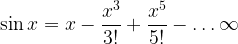$\sin x = x - \dfrac{{{x^3}}}{{3!}} + \dfrac{{{x^5}}}{{5!}} - \ldots\infty$ (f)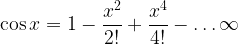$\cos x = 1 - \dfrac{{{x^2}}}{{2!}} + \dfrac{{{x^4}}}{{4!}} - \ldots\infty$ (g)$\tan x = x + \dfrac{{{x^3}}}{3} + \dfrac{{2{x^5}}}{{15}} + \dfrac{{11{x^7}}}{{315}} + \ldots\infty$ (h)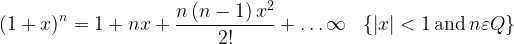${\left( {1 + x} \right)^n} = 1 + nx + \dfrac{{n\left( {n - 1} \right){x^2}}}{{2!}} +\ldots\infty \,\,\,\,\left\{ {\left| x \right| < 1 \,{\rm{and}}\, n\varepsilon Q} \right\}$

(i)
If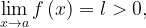$\mathop {\lim }\limits_{x \to a} f\left( x \right) = l > 0,$ then we may writeNote:
We finally need to consider one last important form of limits, namely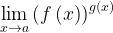$\mathop {\lim }\limits_{x \to a} {\left( {f\left( x \right)} \right)^{g\left( x \right)}}$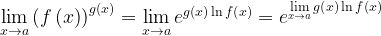$\mathop {\lim }\limits_{x \to a} {\left( {f\left( x \right)} \right)^{g\left( x \right)}} = \mathop {\lim }\limits_{x \to a} {e^{g\left( x \right)\ln f\left( x \right)}} = {e^{\mathop {\lim }\limits_{x \to a} g\left( x \right)\ln f\left( x \right)}}$
(ii) If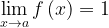$\mathop {\lim }\limits_{x \to a} f\left( x \right) = 1$ then let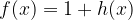$f(x) = 1 + h(x)$. where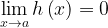$\mathop {\lim }\limits_{x \to a} h\left( x \right) = 0$
Now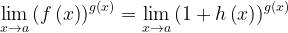$\mathop {\lim }\limits_{x \to a} {\left( {f\left( x \right)} \right)^{g\left( x \right)}} = \mathop {\lim }\limits_{x \to a} {\left( {1 + h\left( x \right)} \right)^{g\left( x \right)}}$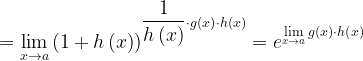$= \mathop {\lim }\limits_{x \to a} {\left( {1 + h\left( x \right)} \right)^{\dfrac{1}{{h\left( x \right)}} \cdot g\left( x \right) \cdot h\left( x \right)}} = {e^{\mathop {\lim }\limits_{x \to a} g\left( x \right) \cdot h\left( x \right)}}$